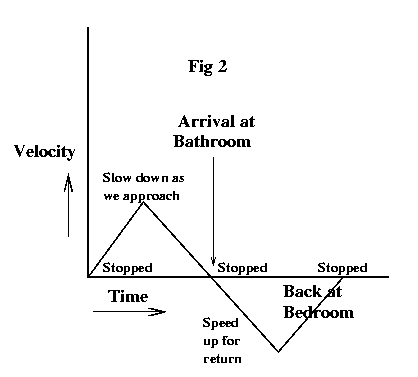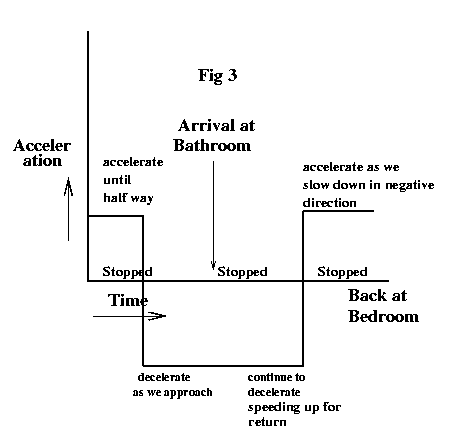Let us begin. We are going to need quite a bit of background, starting with the ideas of distance, velocity, and acceleration. I'm sure we all have a good intuitive idea of these concepts, at least distance and velocity, but acceleration might be a little fuzzy. We need to examine these concepts in the context of something that moves back and forth in a regular motion. Let's do the following "thought experiment." Imagine yourself walking back and forth between your bedroom and your bathroom. As soon as you get to one, you turn around and walk back to the other, and you repeat this over and over again. If we graph the distance you are from your bedroom against time, we get a graph like the one in Fig 1. You'll notice that the distance from the bedroom increases as we approach the bathroom, and decreases as we walk back to the bedroom. Now the definition if velocity is "the rate of change of distance." This makes sense, if our distance from the bedroom changes more quickly, we must be walking faster. Similarly, acceleration is the "rate of change of velocity." If we are speeding up more and more, the acceleration must be getting larger. Now the graph in Fig. 1 isn't very realistic, because when we reach the bathroom and the bedroom, we "instantaneously" turn around and start walking with the opposite velocity in the other direction. What would be more realistic is the graph in Fig. 2 of the velocity versus time during our walk. At the bedroom our initial velocity is 0, we are standing still. Then as we walk towards the bathroom we accelerate, until we get about halfway to the bathroom. Then, realizing that we are approaching and need to turn around, we slow down and when we reach the bathroom we stop and turn around, repeating the process in the opposite direction. Fig. 3 shows the graph of the acceleration that goes with the velocity in Fig. 2. As you can see, we accelerate until we are halfway to the bathroom, then we decelerate, until we stop. Then we continue decelerating, (actually accelerating in the opposite direction) until we are halfway between the bathroom and the bedroom, at which point we start slowing down our "negative velocity" and hence accelerating again, until we reach the bedroom and are ready to repeat the process.

Okay, now let's ask the question, suppose be make twice as many trips back and forth between the bedroom and the bathroom in the same amount of time. Well, we will be covering twice as much distance in the same amount of time, so our velocity will have to be twice as big, in other words we have to walk faster. Now our velocity is going to have to change twice as quickly, so we have to accelerate twice as fast. Thus if we double the "frequency" of our trips, our acceleration will have to be 4 times as fast, or the square of the frequency. This is a fundamental fact that we will need later. Let's restate it so that you'll remember it. Every periodic motion, like you walking back and forth between two points, has a frequency, the number of times per second, minute or hour that it occurs. If you increase the frequency, then the velocity is proportional to the frequency, and the acceleration is proportional to the square of the frequency. Physicists like to use the Greek symbol omega for frequency, but we will use w since it looks like omega. We have shown that acceleration a = k * w^2 where k is some constant, that is acceleration is proportional to the square of the frequency.

Fact # 1: a = k * w^2

Now then, the next piece of the puzzle is the very nature of light itself. You may have heard that "light is an electromagnetic wave," but what the hell does that mean? We have all had the experience of walking across a carpet and getting "zapped" when we touch a doorknob. What happened to us is that we accumulated a bunch of electrons as we walked along the carpet, and the zap we felt is the motion of all these electrons suddenly leaving our body and flowing through the doorknob. There is no question that this "electrical force" exists, even though we were not aware of it as we walked over the carpet. Well, light carries with it this same kind of "electric force" though the amount of the force is so small that we don't feel like we are getting zapped every time the sun comes out. Furthermore, in the case of light, this force travels from place to place in the form of a wave. What creates light? It is a fundamental law of nature that light is created whenever a charged particle, like an electron, accelerates. That is what radio stations do. They move electrons up and down their antennas in a particular pattern, thousands or millions of times per second. These accelerating electrons cause light to appear. The light has a frequency that is too low for our eyes to see, but we have invented special devices, called transistor radios, that can readily detect and decode this light. Physicists like to think of something called an "electromagnetic field" that exists everywhere. You can imagine it as a bunch of tiny invisible arrows at every point in spaces. When light passes by a point in space, these arrows get bigger and smaller. This is the electromagnetic wave passing by. Thus we have fact number two, the E, the electric field that accompanies light, is proportional to a, the acceleration of a charged particle, like an electron.

Fact # 2: E = k * a

We are getting closer now. The next question we have to ask ourselves is "What is it we see?" I know it sounds like a stupid question, but the answer may surprise you, we see "Energy." But what is energy? The real answer is that no one really knows, but physicists have invented elaborate formulas for calculating energy and discovered an amazing fact. If you carefully calculate the energy in a system around you, it never changes, that is energy is conserved. Energy has many different forms and comes in many different disguises. Some examples include kinetic energy, which is the energy that an object has because it is moving. You may remember from high school the formula 1/2 m v^2, for kinetic energy. It says that if an object has mass m and is moving with velocity v, its energy is given by the above formula. Another kind of energy is potential energy. If you lift a brick up in the air, you have given it potential energy, since if you dropped it it would start moving and gain "kinetic energy." There is chemical energy in batteries, and nuclear energy is responsible for our bright sunny days. You won't be surprised to learn that light too carries energy, and if you work out the numbers you will find that the amount of energy that light carries is proportional to the square of the electric field. This is reasonable because the electric field causes charged particles to move, and their velocity will be proportional to the strength of the field. Hence the kinetic energy that they can transfer to these particles is proportional to v^2, the velocity squared, thus the energy of the field itself should also be proportional to E^2. This is more of a definition than a proof, but it is in fact true. Physicists often use the letter S for the energy of light, so we have fundamental fact number 3. The energy of light S, is proportional to the square of the electric field.

Fact # 3: S = k * E^2

What ties this all together? If you look directly at the sun (not a good idea) you see white, which is the color of the light that the sun radiates. If you look at right angles to the sun, you see the light that the air in the atmosphere scatters as the light strikes the air molecules. The light from the sun hits the molecules of the atmosphere and causes their electrons (charged particles) to move back and forth. This back and forth motion of the electrons causes light to created, and it eventually reaches your eyes. This process is known as scattering, and was mathematically explained by Lord Rayleigh back in the 19th century.

Well, we are almost home now. Fact 3 says that energy has that which we see, S, is proportional to the E^2, the electric field squared. Fact 2 says that the electric field E, is proportional to the acceleration of a charged particle. Fact 1 says that the acceleration of an object that moves back and forth, is proportional to the square of the frequency of its motion, a = k w^2. Putting these together we get S = k E^2 = k a^2 = k (w^2)^2 = k w^4. Thus the energy of light that hits our eyes from the air molecules in the atmosphere depends on the fourth power of the frequency of the light. Now it turns out that the frequency of blue light is about twice the frequency of red light, so the air in the atmosphere scatters 2^4 = 16 times more blue light than red light.

Well, we have come a long way, and I'm sure most of you are ready to go home and never look at the sky again, but unfortunately what we have done so far is explain why the sky is violet. What? Yes, violet. You see, if you look at any rainbow you will see that the last color in the rainbow, the one with the highest frequency that we can see, is violet, not blue. Where have we gone wrong? Well, it turns out our eyes are not quite as simple as you might expect. On the back of our eyes are three different kind of cone cells that are responsible for determining the colors that we see. These cells respond to light of different colors in different ways. There are three kinds of cone cells, which respond to red, green, and blue light. The cones that respond to blue light are more sensitive than those that respond to red and green. Furthermore, the indigo and violet that is present in the scattered light stimulate the red and green cones almost equally. Thus what we see is a light shade of blue: blue because our blue cones are the most strongly stimulated, and light because the red and green components, combined with the blue, make white, which lightens the color of the blue that we perceive.

Well, gentle reader, that is the end of the story. I wonder, is this what your father told you when you were a child and asked him, "Why is the sky blue, daddy?"

Quote of the day:
The reason a dog has so many friends is that he wags his tail instead of his tongue.
Anonymous

Sitemap